﻿ 4pqn阶3度对称图的分类 A Classification on Cubic Symmetric Graphs of Order 4pqn

Pure Mathematics
Vol. 09  No. 10 ( 2019 ), Article ID: 33290 , 7 pages
10.12677/PM.2019.910139

A Classification on Cubic Symmetric Graphs of Order 4pqn

Chao Wang

School of Statistics and Mathematics, Yunnan University of Finance and Economics, Kunming Yunnan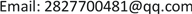Received: Nov. 14th, 2019; accepted: Nov. 27th, 2019; published: Dec. 4th, 2019ABSTRACT

Let $\Gamma$ be a connected graph and $G\le Aut\left(\Gamma \right)$ . Then $\Gamma$ is called a G-basic graph, if G is quasiprimitive or bi-quasiprimitive on $V\Gamma$ . In this paper, we determine cubic symmetric G-basic graphs of order $4p{q}^{n}$ , where $p are odd primes, and $n\ge 1$ .

Keywords:Symmetric Graph, Locally Primitive, Quasiprimitive, Bi-Quasiprimitive, Almost Simple4pqn阶3度对称图的分类$\Gamma$ 是一个连通图， $G\le Aut\left(\Gamma \right)$，如果G作用在 $V\Gamma$ 上是拟本原或顶点二部拟本原的，则称 $\Gamma$ 是G-基图。在本文中，我们将 $4p{q}^{n}$ 阶3度对称G-基图，其中 $p 为奇素数， $n\ge 1$1. 引言

$G\le \text{Sym}\left(\Omega \right)$ 是一个传递置换群，如果G的每个极小正规子群在 $\Omega$ 上都是传递的，则称G是拟本原的；如果G的每个极小正规子群在 $\Omega$ 上至多有两个轨道且存在一个极小正规子群在 $\Omega$ 上恰好有两个轨道，则称G是二部拟本原的。对于一个图 $\text{Γ}$$G\le \text{Aut}\left(\text{Γ}\right)$，如果G在顶点集 $V\text{Γ}$ 上是拟本原或二部拟本原的，则称 $\text{Γ}$ 是一个G-基图。研究对称图的方法分为以下两步：Table 1. G-basic symmetric graphs of order 4 p q n and valency 3

2. 预备知识Table 2. Point-stabilizer groups of cubic symmetric groups

1) N在 $V\Gamma$ 上半正则， $G/N\le \text{Aut}\left({\Gamma }_{N}\right)$${\Gamma }_{N}$$G/N$ -弧传递的且 $\text{Γ}$${\Gamma }_{N}$ 的正规N-覆盖；

2) $\text{Γ}$$\left(G,s\right)$ -弧传递的当且仅当 $\left(G/N,s\right)$ -弧传递的，其中 $1\le s\le 5$$s=7$

3) ${G}_{\alpha }\cong {\left(G/N\right)}_{\delta }$，其中 $\alpha \in V\text{Γ}$$\delta \in V{\Gamma }_{N}$

HA (仿射型)： $\text{soc}\left(X\right)$ 是交换的，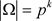，其中p是素数，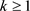HS (全形单型)：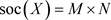，其中 $N\cong M\cong {T}^{}$

HC (复合全型)： $\text{soc}\left(X\right)=M×N$，其中 $N\cong M\cong {T}^{k}$

AS (几乎单型)： $\text{soc}\left(X\right)=T$ 是非交换单群， $T⊲X\le \text{Aut}\left(T\right)\le T.\text{Out}\left(T\right)$

TW (扭圈积型)：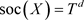$\Omega$ 上正则， $|\Omega |={|T|}^{k}$，其中 $d\ge 2$

SD (单对角型)： $\text{soc}\left(X\right)={T}^{k}$$\text{soc}{\left(X\right)}_{\alpha }=T$$|\Omega |={|T|}^{k-1}$，其中 $d\ge 2$$\alpha \in \Omega$

CD (复合对角型)： $\text{soc}\left(X\right)={T}^{k}$$\text{soc}{\left(X\right)}_{\alpha }={T}^{l}$$|\Omega |={|T|}^{k-l}$，其中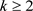$l|k$$\alpha \in \Omega$

PA (乘积作用型)： $\text{soc}\left(X\right)={T}^{r}$$\text{soc}{\left(X\right)}_{\alpha }\ne 1$，其中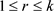$\alpha \in \Omega$

(2) $G=\text{PSL}\left(\text{2},\text{11}\right)$，则G存在子群同构于 ${\text{S}}_{3}$，此时存在一个阶为220阶的3度对称图，记为$\text{Aut}\left(\text{Γ}\right)\cong \text{PSL}\left(2,11\right)$

(2) $G=\text{PSL}\left(2,13\right)$，则G存在子群同构于 ${\text{S}}_{3}$，此时存在一个阶为364阶的3度对称图，记为 ${\mathcal{G}}_{364}^{2}$$\text{Aut}\left(\text{Γ}\right)\cong \text{PSL}\left(2,13\right)$

3. 定理1.1的证明

${2}^{7}\nmid |T|,\text{\hspace{0.17em}}\text{\hspace{0.17em}}{3}^{2}\nmid |T|,\text{\hspace{0.17em}}\text{\hspace{0.17em}}{r}^{2}\nmid |T|$(1)

$\frac{1}{2}\left(q-1\right)\left(q+1\right)|{2}^{6}\cdot 3\cdot r$

$\frac{q-1}{2}\cdot \frac{q+1}{2}|{2}^{5}\cdot 3\cdot r$Table 3. Nonabelian groups with 3 prime factors and ( p , q n )Table 4. Nonabelian groups with 4 prime factors and ( p , q n )

1) ${G}^{+}$${\Delta }_{i}$ 上是拟本原的；或

2) ${G}^{+}$ 中存在两个正规子群 ${M}_{1}$，使得 ${M}_{1}\cong {M}_{2}$ 且在 $V\Gamma$ 上半正则。进一步地，有群 $M={M}_{1}×{M}_{2}$${\Delta }_{i}$ 上是正则的。

$|\pi \left(T\right)|=3$ 时，则T和 $\left(p,{q}^{n}\right)$ 满足表3。如果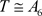，则 $|{T}_{\alpha }|=|T|/|{\Delta }_{i}|=12$。因为 $\text{Out}\left({\text{A}}_{6}\right)={ℤ}_{2}^{2}$，所以 $o={ℤ}_{2}$${o}^{\prime }=1$$o={ℤ}_{2}^{2}$${o}^{\prime }={ℤ}_{2}$。故 $|{G}_{\alpha }|=|{G}_{\alpha }^{+}|=|{T}_{\alpha }|\cdot |{o}^{\prime }|=12$ 或 ， $\text{G}\cong {\text{S}}_{6}$$\text{Aut}\left({\text{A}}_{6}\right)$。由Magma (  )，这两种情况下都没有图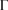存在。如果 $T\cong \text{PSL}\left(2,8\right)$，因为 $\text{Out}\left(\text{PSL}\left(2,8\right)\right)={ℤ}_{3}$ 没有指数为2的子群，与 $|o:{o}^{\prime }|=2$ 矛盾。如果 $T\cong \text{PSL}\left(2,17\right)$，因为 $\text{Out}\left(\text{PSL}\left(2,17\right)\right)={ℤ}_{2}$$|{T}_{\alpha }|=|T|/|{\Delta }_{i}|=24$，所以 $|{G}_{\alpha }|=|{G}_{\alpha }^{+}|=|{T}_{\alpha }|\cdot |{o}^{\prime }|=24$$G\cong \text{PSL}\left(2,17\right).{ℤ}_{2}=\text{PGL}\left(2,17\right)$，由Magma (  )，不存在满足条件的图 $\Gamma$

$|\pi \left(T\right)|=4$ 时， $\left(T,p,{q}^{n}\right)$ 满足表4。如果 $T\cong \text{PSL}\left(2,193\right)$，则 $|{T}_{\alpha }|=|T|/|{\Delta }_{i}|=96$，从而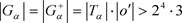。这与定理2.1矛盾。如果 $T\cong \text{PSL}\left(2,32\right)$，则 $\text{Out}\left(T\right)={ℤ}_{5}$，因为 $|o:{o}^{\prime }|=2$，矛盾。如果 $T\cong \text{PSL}\left(2,{2}^{4}\right)$，则 $|{T}_{\alpha }|=|T|/|{\Delta }_{i}|=24$。因为，故 $G\cong \text{PGL}\left(2,16\right)$$\text{Aut}\left(\text{PSL}\left(2,16\right)\right)$，且对应的 ${G}_{\alpha }$ 分别为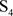${\text{S}}_{4}×{ℤ}_{2}$，由Magma (  )可知 $\text{PGL}\left(2,{2}^{4}\right)$ 中没有24阶子群，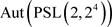中没有同构于 ${\text{S}}_{4}×{ℤ}_{2}$ 的48阶子群，矛盾。如果 $T\cong \text{PSL}\left(2,31\right)$，则 $|{T}_{\alpha }|=48$。又因为 $\text{Out}\left(\text{PSL}\left(2,31\right)\right)={ℤ}_{2}$，所以 $G\cong \text{PGL}\left(2,31\right)$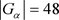。与 $\text{PGL}\left(2,31\right)$ 中没有48阶子群矛盾。若 $T\cong \text{PSL}\left(2,23\right)$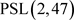时，由Magma (  )，不存在这样的3度对称图。若 $T\cong \text{PSL}\left(2,11\right)$$\text{PSL}\left(2,13\right)$，由例2.4和2.5可知， $\text{Γ}\cong {\mathcal{G}}_{220}^{2}$${\mathcal{G}}_{364}^{2}$

A Classification on Cubic Symmetric Graphs of Order 4pqn[J]. 理论数学, 2019, 09(10): 1132-1138. https://doi.org/10.12677/PM.2019.910139

1. 1. Chao, C.Y. (1971) On the Classification of Symmetric Graphs with a Prime Number of Vertices. Transactions of the American Mathematical Society, 158, 247-256. https://doi.org/10.2307/1995785

2. 2. Cheng, Y. and Oxley, J. (1987) On Weakly Symmetric Graphs of Order Twice a Prime. Journal of Combinatorial Theory, Series B, 42, 196-211. https://doi.org/10.1016/0095-8956(87)90040-2

3. 3. Wang, R.J. and Xu, M.Y. (1993) A Classification of Symmetric Graphs of Order 3p. Journal of Combinatorial Theory, Series B, 58, 197-216. https://doi.org/10.1006/jctb.1993.1037

4. 4. Feng, Y.Q. and Kwak, J.H. (2006) Cubic Symmetric Graphs of Order Twice an Odd Prime Power. Journal of the Australian Mathematical Society, 81, 153-164. https://doi.org/10.1017/S1446788700015792

5. 5. Feng, Y.Q. and Kwak, J.H. (2007) Cubic Symmetric Graphs of Or-der a Small Number Times a Prime or a Prime Square. Journal of Combinatorial Theory, Series B, 97, 627-646. https://doi.org/10.1016/j.jctb.2006.11.001

6. 6. Feng, Y.Q., Zhou, J.X. and Li, Y.T. (2016) Pentavalent Symmetric Graphs of Order Twice a Prime Power. Discrete Mathematics, 339, 2640-2651. https://doi.org/10.1016/j.disc.2016.05.008

7. 7. Hua, X.H., Chen, L. and Xiang, X. (2018) Valency Seven Symmetric Graphs of Order 2pq. Czechoslovak Mathematical Journal, 68, 581-599. https://doi.org/10.21136/CMJ.2018.0530-15

8. 8. Lu, Z.P., Wang, C.Q. and Xu, M.Y. (2004) On Semisymmetric Cubic Graphs of Order 6p2. Science in China Series A Mathematics, 47, 1-17.

9. 9. Zhou, J.X. and Feng, Y.Q. (2010) Tetravalent s-Transitive Graphs of Order Twice a Prime Power. Journal of the Australian Mathematical Society, 88, 277-288. https://doi.org/10.1017/S1446788710000066

10. 10. Tutte, W.T. (1947) A Family of Cubical Graphs. Mathematical Proceedings of the Cambridge Philosophical Society, 43, 459-474. https://doi.org/10.1017/S0305004100023720

11. 11. Zhou, J.X. and Feng, Y.Q. (2010) Cubic Vertex-Transitive Graphs of Order 2pq. Journal of Graph Theory, 65, 285-302. https://doi.org/10.1002/jgt.20481

12. 12. Huppert, B. and Lempken, W. (2000) Simple Groups of Order Divisible by at Most Four Primes. Francisk Skorina Gomel State University, 16, 64-75.

13. 13. 徐明耀. 有限群导引(上, 下)[M]. 北京: 科学出版社, 1999.

14. 14. Dixon, J. and Mortimer, D.B. (1997) Permutation Groups. Spring-Verlag, New York. https://doi.org/10.1007/978-1-4612-0731-3

15. 15. Li, C.H. and Pan, J.M. (2008) Finite 2-Arc-Transitive Abelian Cayley Graphs. European Journal of Combinatorics, 29, 148-158. https://doi.org/10.1016/j.ejc.2006.12.001

16. 16. Praeger, C.E. (1992) An O’Nan-Scott Theorem for Finite Quasiprimitive Permutation Groups and an Application to 2-Arc-Transitive Graphs. Journal of the London Mathematical Society, 47, 227-239. https://doi.org/10.1112/jlms/s2-47.2.227

17. 17. Bosma, W., Cannon, J. and Playoust, C. (1997) The MAGMA Algebra System I: The User Language. Journal of Symbolic Computation, 24, 235-265. https://doi.org/10.1006/jsco.1996.0125

18. 18. Conder, M. and Dobcsabyi, P. (2002) Trivalent Symmetric Graphs on up to 768 Vertices. Journal of Combinatorial Mathematics and Combinatorial Computing, 40, 41-63.

19. 19. Giudici, M., Li, C.H. and Praeger, C.E. (2003) Analysing Finite Locally s-Arc-Transitive Graphs. Transactions of the American Mathematical So-ciety, 356, 291-317. https://doi.org/10.1090/S0002-9947-03-03361-0

20. 20. Li, C.H., Praeger, C.E., Venkatesh, A. and Zhou, S.M. (2002) Finite Locally-Quasiprimitive Graphs. Discrete Mathematics, 246, 197-218. https://doi.org/10.1016/S0012-365X(01)00258-8